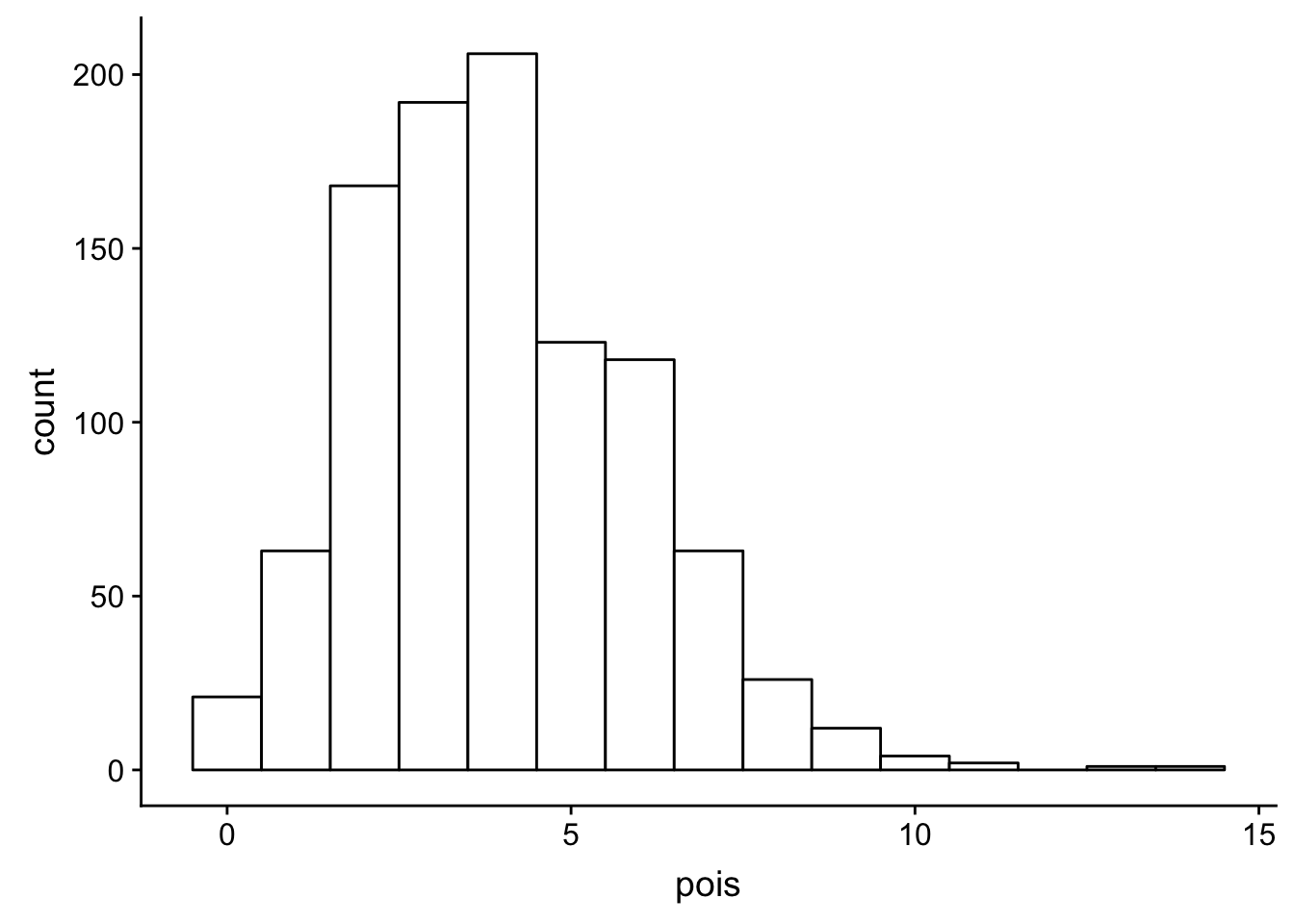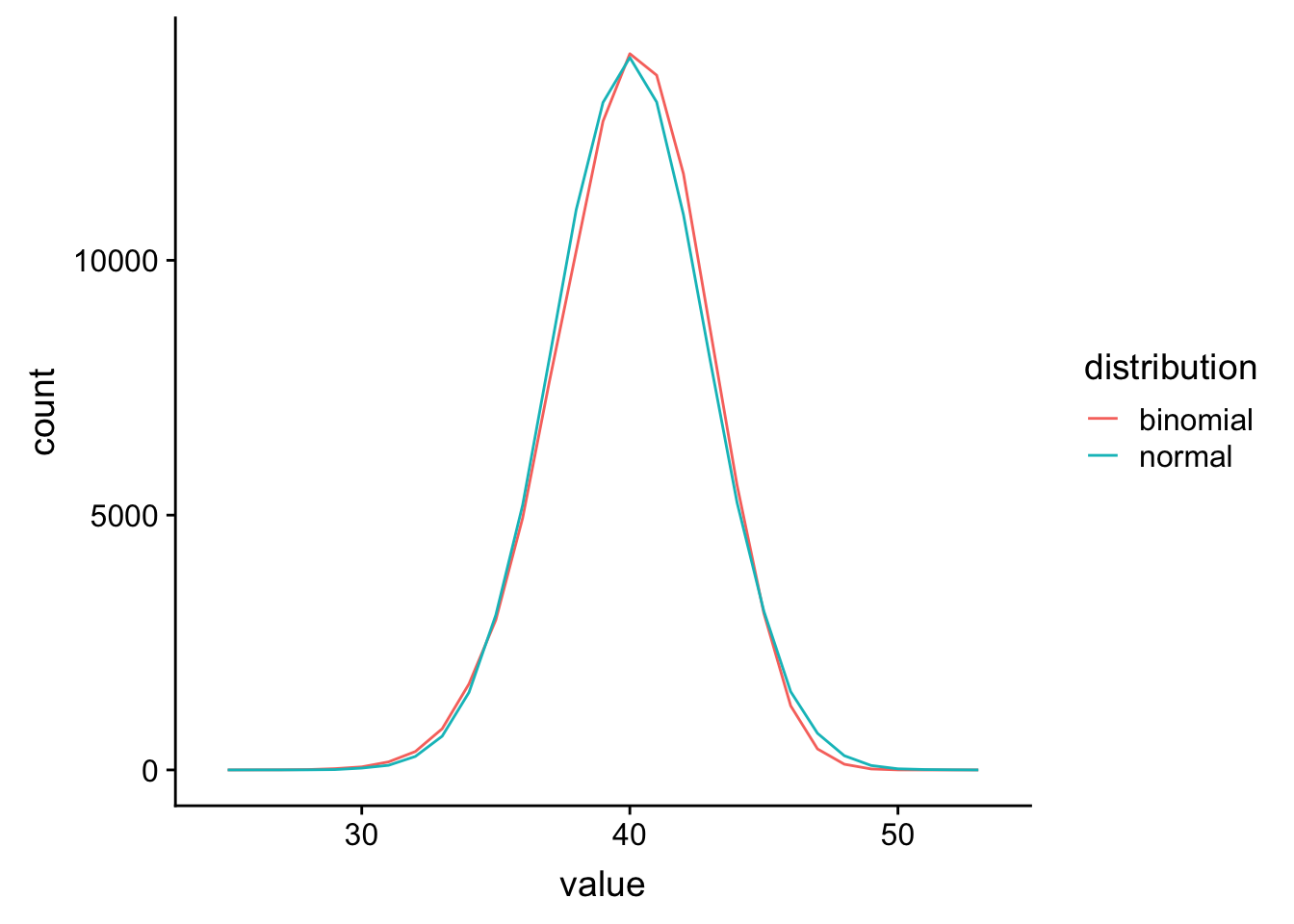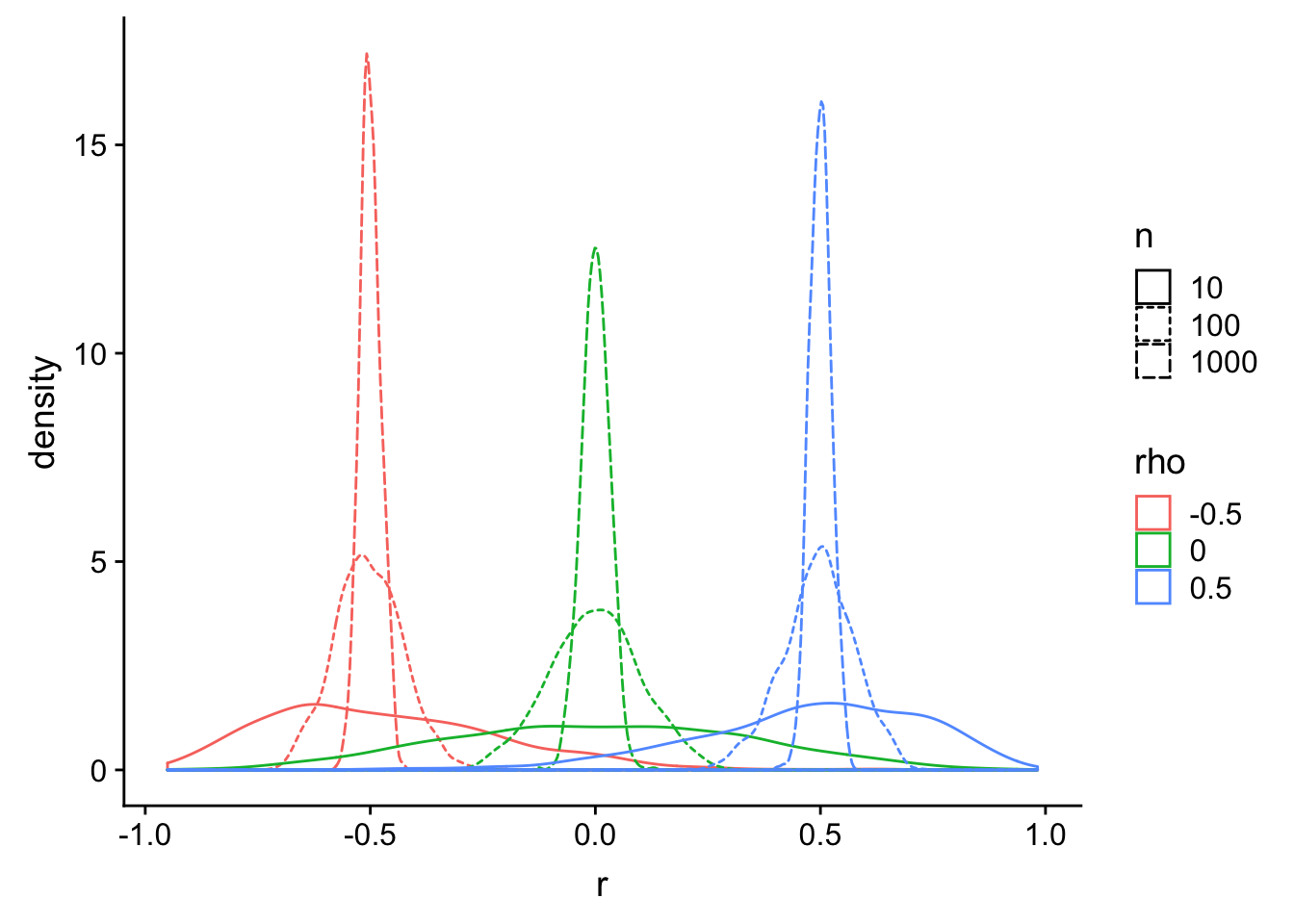## Generate Data

### Question 1

Generate 50 data points from a normal distribution with a mean of 0 and SD of 1.

a <- rnorm(50, 0, 1)

### Question 2

Generate another variable (b) that is equal to the sum of a and another 50 data points from a normal distribution with a mean of 0.5 and SD of 1.

b <- a + rnorm(50, 0.5, 1)

### Question 3

Run a two-tailed, paired-samples t-test comparing a and b.

t <- t.test(a, b, paired = TRUE)

t # prints results of t-test
##
##  Paired t-test
##
## data:  a and b
## t = -3.4945, df = 49, p-value = 0.001018
## alternative hypothesis: true difference in means is not equal to 0
## 95 percent confidence interval:
##  -0.9500592 -0.2563093
## sample estimates:
## mean of the differences
##              -0.6031843

## Calculate Power

Use at least 1e4 replications to estimate power accurately.

### Question 4

Calculate power for a two-tailed t-test with an alpha (sig.level) of .05 for detecting a difference between two independent samples of 50 with an effect size of 0.3.

Hint: You can use the sim_t_ind function from the T-Test Class Notes.

sim_t_ind <- function(n, m1, sd1, m2, sd2) {
v1 <- rnorm(n, m1, sd1)
v2 <- rnorm(n, m2, sd2)
t_ind <- t.test(v1, v2, paired = FALSE)

return(t_ind$p.value) } my_reps <- replicate(1e4, sim_t_ind(50, 0, 1, 0.3, 1)) alpha <- 0.05 power.sim <- mean(my_reps < alpha) power.sim # prints the value ##  0.3253 ### Question 5 Compare this to the result of power.t.test for the same design. power.analytic <- power.t.test(n = 50, delta = 0.3, sig.level = 0.05, type = "two.sample")$power

power.analytic  # prints the value
##  0.3175171

### Question 6

Modify the sim_t_ind function to handle different sample sizes. Use it to calculate the power of the following design:

• 20 observations from a normal distribution with mean of 10 and SD of 4 versus
• 30 observations from a normal distribution with a mean of 13 and SD of 4.5
• alpha (sig.level) of 0.05
sim_t_ind <- function(n1, m1, sd1, n2, m2, sd2,
alternative = "two.sided") {
v1 <- rnorm(n1, m1, sd1)
v2 <- rnorm(n2, m2, sd2)
t_ind <- t.test(v1, v2,
alternative = alternative,
paired = FALSE)

return(t_ind$p.value) } my_reps <- replicate(1e4, sim_t_ind(20, 10, 4, 30, 13, 4.5)) power6 <- mean(my_reps < 0.05) power6 # prints the value ##  0.6789 ### Question 7 Do noisy environments slow down reaction times for a dot-probe task? Calculate power for a one-tailed t-test with an alpha (sig.level) of .005 for detecting a difference of at least 50ms, where participants in the quiet condition have a mean reaction time of 800ms. Assume both groups have 80 participants and both SD = 100. my_reps <- replicate(1e4, sim_t_ind(80, 800, 100, 80, 850, 100, "less")) power7 <- mean(my_reps < 0.005) power7 # prints the value ##  0.7069 ## Poisson Distribution The [poisson distribution(https://en.wikipedia.org/wiki/Poisson_distribution) is good for modeling the rate of something, like the number of texts you receive per day. Then you can test hypotheses like you receive more texts on weekends than weekdays. The poisson distribution gets more like a normal distribution when the rate gets higher, so it’s most useful for low-rate events. ### Question 8 Use ggplot to create a histogram of 1000 random numbers from a poisson distribution with a lambda of 4. lambda <- 4 # lambda sets the mean of the poisson distribution pois <- rpois(1000, lambda) ggplot() + geom_histogram(aes(pois), fill="white", color="black", binwidth = 1)## Intermediate: Binomial Distribution ### Question 9 Demonstrate to yourself that the binomial distribution looks like the normal distribution when the number of trials is greater than 10. Hint: You can calculate the equivalent mean for the normal distribution as the number of trials times the probability of success (binomial_mean <- trials * prob) and the equivalent SD as the square root of the mean times one minus probability of success (binomial_sd <- sqrt(binomial_mean * (1 - prob))). n <- 1e5 # use a large n to get good estimates of the distributions trials <- 50 prob <- 0.8 binomial_mean <- trials * prob binomial_sd <- sqrt(binomial_mean * (1 - prob)) # sample numbers from binomial and normal distributions norm_bin_comp <- tibble ( normal = rnorm(n, binomial_mean, binomial_sd), binomial = rbinom(n, trials, prob) ) %>% gather("distribution", "value", normal:binomial) # plot the sampled numbers to compare ggplot(norm_bin_comp, aes(value, color=distribution)) + geom_freqpoly(binwidth = 1)## Advanced: Correlation power simulation Remember, there are many, many ways to do things in R. The important thing is to create your functions step-by-step, checking the accuracy at each step. ### Question 10 Write a function to create a pair of variables of any size with any specified correlation. Have the function return a tibble with columns x and y. Make sure all of the arguments have a default value. Hint: modify the code from the Bivariate Normal section from the class notes. bvn2 <- function(n = 10, rho = 0, m1 = 0, m2 = 0, sd1 = 1, sd2 = 1) { # n = number of random samples # rho = population correlation between the two variables mu <- c(m1, m2) # the means of the samples stdevs <- c(sd1, sd2) # the SDs of the samples # correlation matrix cor_mat <- matrix(c( 1, rho, rho, 1), 2) # create the covariance matrix sigma <- (stdevs %*% t(stdevs)) * cor_mat # sample from bivariate normal distribution m <- mvrnorm(n, mu, sigma) # convert to a tibble to make it easier to deal with in further steps colnames(m) <- c("x", "y") as_tibble(m) } bvn2() %>% knitr::kable() # prints the table for default arguments x y -1.0870058 0.9854768 -0.0677941 -1.5955569 -0.6553285 -0.1398619 -1.0965979 0.0318778 1.0129517 -2.5729905 3.3090125 0.7315682 -0.3829692 0.3183240 1.0167431 0.2253734 1.3085627 1.1144965 0.7117681 -1.1009135 ### Question 11 Use cor.test to test the Pearson’s product-moment correlation between two variables generated with your function having an n of 50 and a rho of 0.45. my_vars <- bvn2(50, 0.45) cor.test(my_vars$x, my_vars$y) ## ## Pearson's product-moment correlation ## ## data: my_vars$x and my_vars$y ## t = 3.5145, df = 48, p-value = 0.0009723 ## alternative hypothesis: true correlation is not equal to 0 ## 95 percent confidence interval: ## 0.1991195 0.6490155 ## sample estimates: ## cor ## 0.4523935 ### Question 12 Test your function from Question 10 by calculating the correlation between your two variables many times for a range of values and plotting the results. Hint: the purrr::map() functions might be useful here. # set up all values you want to test sims <- crossing( rep = 1:1000, # how many replications per combination n = c(10, 100, 1000), rho = c(-0.5, 0, .5) ) %>% mutate(r = purrr::map2_dbl(n, rho, function(n, rho) { b <- bvn2(n = n, rho = rho) cor(b$x, b$y) }), n = as.factor(n), # factorise for plotting rho = as.factor(rho) # factorise for plotting ) ggplot(sims, aes(r, color=rho, linetype=n)) + geom_density()### Question 13 Make a new function that calculates power for cor.test through simulation. power.cor.test <- function(reps = 1000, n = 50, rho = 0.5, alpha = 0.05, method = "pearson"){ power <- tibble( data = rerun(reps, bvn2(n, rho)) ) %>% mutate(power = map(data, function(data) { cor.test(data$x, data$y, method = method) %>% broom::tidy() })) %>% unnest(power) %>% mutate(sig = p.value < alpha) tibble( n = n, rho = rho, alpha = alpha, power = mean(power$sig),
method = method,
reps = reps
)
}

power.cor.test(1000, 30, 0.5, method = "spearman") %>%
knitr::kable()
n rho alpha power method reps
30 0.5 0.05 0.764 spearman 1000